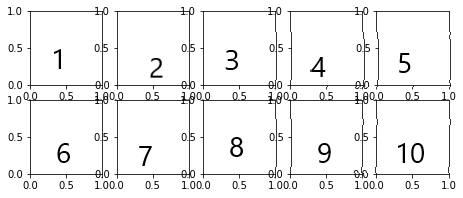# Defining some Utility Functions

• We shall define 3 utility functions which we will be using further:

• `rounded_accuracy`: we define this function to get the rounded accuracy by calculating the accuracies using the rounded values of the predicted value and the actual value.

• `plot_image` : used to plot the given image

• `show_reconstructions` : we shall use this function to show how our trained model is able to reconstruct the validation data.

Note:

• `keras.metrics.binary_accuracy` : calculates how often predictions matches binary labels. It computes the mean accuracy rate across all predictions for binary classification problems.

• `plt.imshow` : Display data as an image

• `plt.axis("off")` : It does not display the axis.

• `plt.subplot` : Add a subplot to the current figure.

INSTRUCTIONS
• Define the `rounded_accuracy`:

``````def rounded_accuracy(y_true, y_pred):
return keras.metrics.binary_accuracy(tf.round(y_true), tf.round(y_pred))
``````
• Define the `plot_image` function:

``````def plot_image(image):
plt.imshow(image, cmap="binary")
plt.axis("off")
``````
• Define the `show_reconstructions`:

``````def show_reconstructions(model, images=X_valid, n_images=5):
reconstructions = model.predict(images[:n_images])
fig = plt.figure(figsize=(n_images * 1.5, 3))
for image_index in range(n_images):
plt.subplot(2, n_images, 1 + image_index)
plot_image(images[image_index])
plt.subplot(2, n_images, 1 + n_images + image_index)
plot_image(reconstructions[image_index])
``````

Here, we are doing the following:

1. we are getting the reconstructions as predicted by the model.
2. we are creating a matplotlib figure object and setting its size to (n_images * 1.5, 3), ie (5 * 1.5, 3)
3. Nextly, for each of the n_images, we are creating the subplots and displaying the images in those subplots: the first row will display actual images and the second row will display the predicted images. The indices are set accordingly. Please have a look at the below image and check the indices used in the subplots to get a better understanding:No hints are availble for this assesment

Answer is not availble for this assesment

Note - Having trouble with the assessment engine? Follow the steps listed here